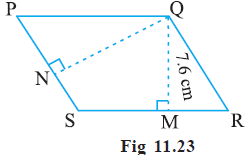# Ex.11.2 Q5 Perimeter and Area - NCERT Maths Class 7

Go back to  'Ex.11.2'

## Question

$$PQRS$$ is a parallelogram (Fig 11.23). $$QM$$ is the height from $$\, Q \,$$ to $$SR$$ and $$QN$$ is the height from$$\, Q \,$$ to $$PS$$. If $$SR$$ $$= 12\,\rm cm$$ and $$QM$$ $$= 7.6 \,\rm cm$$.

Find:

(a) the area of the parallelogram $$PQRS$$

(b) $$QN$$, if $$PS$$ $$= 8 \,\rm cm$$## Text Solution

What is known?

$$PQRS$$ is a parallelogram. $$QM$$ is the height from $$\, Q \,$$ to $$SR$$ and $$QN$$ is the height from $$\, Q \,$$ to $$PS$$. Also, $$SR$$ $$= 12\,\rm cm$$ and $$QM$$ $$= 7.6 \,\rm cm$$.

What is unknown?

(a) The area of the parallelogram $$PQRS$$ and (b) $$QN$$, if $$PS$$ $$= 8 \,\rm cm$$

Reasoning:

First, using the given information (base $$SR$$ = 12cm and perpendicular height $$QM$$ = 7.6cm), find out the area of parallelogram $$PQRS$$. Now, area of $$PQRS$$ and base $$PS$$ is known. Again, by using the formula of area of parallelogram, find out height $$QN$$.

Steps:

(a) Given, $$SR$$ $$= 12\,\rm cm$$ and

$$QM$$ $$= 7.6 \,\rm cm$$.

\begin{align} & \text{Area of parallelogram} \; PQRS \\ &= {\rm{Base}} \times {\rm{Height}} \end{align}

\begin{align}&= SR \times QM \\&= 12 \times 7.6\\&= 91.2 \, \rm cm^2\end{align}

(b) Given, Base $$PS$$ $$= 8 \,\rm cm$$

Area of the parallelogram $$= 91.2 \rm\, cm^2$$ [calculated in part (a)]

\begin{align} & \text{Area of parallelogram}\; PQRS \\ &= {\rm{Base}} \times {\rm{Height}} \end{align}

\begin{align} 91.2 &= 8 \times QN \\{{QN}} &= \frac{91.2}{8}\\{{QN}} &= 11.4\;\rm cm\end{align}

Learn from the best math teachers and top your exams

• Live one on one classroom and doubt clearing
• Practice worksheets in and after class for conceptual clarity
• Personalized curriculum to keep up with school